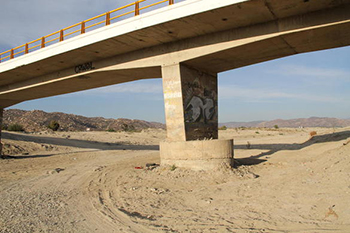online_scour_froehlich1988:  Calculation of pier scour using the Froehlich (1988) formulaFormula:

Fr1 = v1 / (g y1)1/2

ys = 0.32 b φ Fr10.2 (be / b)0.62 (y1 / b)0.46 (b / D50)0.08 + b

[ SI units (metric):  ys, y1, and b in m; v1 in m/s; D50 in mm ]
[ U.S. Customary units:  ys, y1, and b in ft; v1 in ft/s; D50 in mm ]
[ Fr = Froude number; g = gravitational acceleration ]

DESCRIPTION      [Sample Input]     [Main page]

To determine the bridge scour depth ys, the calculator requires the following information:

• Flow depth directly upstream of the pier y1, in m (or ft).

• Mean flow velocity directly upstream of the pier v1, in m/s (or fps).

• Pier width in a horizontal direction normal to the flow b (Fig. 1), in m (or ft).Fig. 1  Definition sketch for pier scour.

• Pier length L (Fig. 1), in m (or ft).

• Angle of attack θ of the flow, in degrees.

• Pier nose shape (Fig. 2), to determine the shape co-efficient φ.

• φ = 1.3 for square-nosed piers.

• φ = 1.0 for round-nosed piers.

• φ = 0.7 for sharp-nosed piers.Fig. 2  Common pier shapes.